• iir数字滤波器matlab程序
千次阅读 多人点赞
2021-01-21 12:58:43

# 一、实验目的

• 熟悉用双线性变换法设计 IIR 数字滤波器的原理与方法
• 学会调用 MATLAB 信号处理工具箱中滤波器设计函数（或滤波器设计分析工具fdatool）设计各种 IIR 数字滤波器， 学会根据滤波需求确定滤波器指标参数。
• 掌握 IIR 数字滤波器的 MATLAB 实现方法
• 通过观察滤波器输入输出信号的时域波形及其频谱，建立数字滤波的概念

# 二、实验原理

设计IIR数字滤波器一般采用间接法（脉冲响应不变法和双线性变换法），应用最广泛的是双线性变换法。

基本设计过程如下：

• 先将给定的数字滤波器的指标转换成过渡模拟滤波器的指标
• 设计过渡模拟滤波器
• 将过渡模拟滤波器的系统函数转换成数字滤波器的系统函数，MATLAB信号处理工具箱中的各种 IIR 数字滤波器设计函数都是采用双线
性变换法。

教材第 6 章介绍的滤波器设计函数butter、 cheby1 、cheby2 和 eclip 可以分别被调用来直接设计巴特沃斯、 切比雪夫1、 切比雪夫 2 以及椭圆模拟和数字滤波器。 本实验要求读者调用如上函数直接设计 IIR 数字滤波器。本实验的数字滤波器的 MATLAB 实现是指调用 MATLAB 信号处理工具箱函数 filter 对给定的输入信号 x(n) 进行滤波，得到滤波后的输出信号y(n)。

原理框图如下：

更多相关内容
• matlab代码，直接运行出结果，含以下几个部分的代码： 1. 巴特沃兹带通滤波器设计 2.带阻滤波器设计 3.递归型滤波器设计 4.对信号进行数字滤波
• MatlabIIR数字滤波器的设计-实验六 IIR数字滤波器的设计.zip IIR数字滤波器的设计的全部程序，拿来与大家共享，大家快来共享哦！！谢谢支持哦
• iir数字滤波器设计及其matlab实现 1IIR 数字滤波器设计及其 MATLAB 实现自动化学院 杨梅 201422070125摘 要IIR 数 字 滤 波 器 在 设 计 上 可 以 借 助 成 熟 的 模 拟 滤 波 器 的 成 果 ，如 巴 特 沃 斯 、 契 ...iir数字滤波器设计及其matlab实现

1IIR 数字滤波器设计及其 MATLAB 实现自动化学院 杨梅 201422070125摘 要IIR 数 字 滤 波 器 在 设 计 上 可 以 借 助 成 熟 的 模 拟 滤 波 器 的 成 果 ，如 巴 特 沃 斯 、 契 比 雪 夫 和 椭 圆 滤 波 器 等 。 在 设 计 一 个 IIR 数 字 滤波 器 时 ， 我 们 根 据 指 标 先 写 出 模 拟 滤 波 器 的 设 计 方 法 设 计 一 个 满足 数 字 滤 波 器 指 标 的 模 拟 滤 波 器 ， 然 后 通 过 一 定 的 变 换 ， 将 模 拟滤 波 器 转 换 成 数 字 滤 波 器 。 本 文 介 绍 了 IIR 数 字 滤 波 器 设 计 ， 主要 分 析 了 其 在 满 足 上 述 要 求 下 的 工 程 方 法 ， 冲 激 响 应 不 变 法 和 双线 性 变 换 法 。 通 过 理 论 与 实 践 的 分 析 对 比 ， 表 明 了 两 种 方 法 的 优弊 端 。关键词 IIR 数字滤波器；M ATLAB； 冲激响应不变法；双线性变换法1. 引言IIR 数字滤波器的最通用的方法是借助于模拟滤波器的设计方法。模拟滤波器设计已经有了相当成熟的技术和方法，有完整的设计公式，还有比较完整的图表可以查询，因此设计数字滤波器可以充分利用这些丰富的资源来进行。对于 IIR 数字滤波器的设计具体步骤： (1)按照一定的规则将给出的数字滤波器的技术指标转换为模拟低通滤波器的技术指标。(2)根据转换后的技术指标设计模拟低通滤波器 G(s)(G(s)是低通滤2波器的传递函数)。(3) 再按照一定的规则将 G(s)转换成 H(z)(H(z)是数字滤波器的传递函数).下面从原理分析在到实践来分析设计用冲激响应不变法和双线性变换法来设计 IIR 数字滤波器的过程。2. 目标分析冲激响应不变法是一种简单的转换方法，它可以将一个稳定的连续时间系统转换为一个稳定的离散时间系统，而且可以使离散时间系统的冲激响应形状和连续时间系统相同，但是，由于连续时间系统的频率响应往往不是限带频谱，因此，这种方法存在着频谱混叠的现象。这种混叠将造成频谱高端的严重失真，从而使得这种方法只适用于低通滤波器或者频谱限带的高通或带通。与脉冲响应不变法相比，双线性变换的主要优点：靠频率的严重非线性关系得到 S 平面与 Z 平面的单值一一对应关系，整个 jΩ 轴单值对应于单位圆一周，这个关系就是式 所表示的，其中 ω 和 Ω为非线性关系。如图图中看到，在零频率附近，Ω～ω 接近于线性关系，Ω 进一步增加时，ω 增长变得缓慢， (ω 终止于折叠频率处) ，所以双线性变换不会出现由于高频部分超过折叠频率而混淆到低频部分去的现象。双线性变换法的缺点：Ω 与 ω 的非线性关系，导致数字滤波器的幅频响应相对于模拟滤波器的幅频响应有畸变，(使数字滤波器与模拟滤波器在响应与频率的对应关系上发生畸变)。下面通过实例来分析两种方法设计 IIR 低通滤波器。3. 实例分析设计低通数字滤波器，要求在通带内频率低于 0.2πrad 时，允许3幅度误差在 1dB 以内，在频率 0.3πrad 到 πrad 之间的阻带衰减大于 15dB。用脉冲响应不变法设计数字滤波器。T＝1。,要求利用-切比雪夫滤波器原型来设计 IIR 低通滤波器。需要对信号做频谱分析，为了简便对比，对信号进行频谱分析时，这里统一采用无纲量的归一化频率单位，即模拟频率对采样频率归一化；模拟角频率对采样角频率归一化；数字频率对 2π 归一化。方法步骤先将技术指标转换为模拟低通滤波器的技术指标，再求出阶数 N，查表，得出模拟低通滤波器 G(s)，按一定规则 G(s)转换成 H(z)，并作出频谱分析的图像，利用 MATLAB 实现上述分析过程的程序如下：冲激响应不变法：wp=0.2*pi;wr=0.3*pi;Ap=1;Ar=15;T=1;Omegap=wp/T;Omegar=wr/T;[cs,ds]=afd_chb1(Omegap,Omegar,Ap,Ar) %切比雪夫 1 型[C,B,A]=sdir2cas(cs,ds); %模拟滤波器级联型[db,mag,pha,Omega]=freqs_m(cs,ds,pi); %模拟滤波器响应subplot(224);plot(Omega/pi,mag);title( 模拟滤波器幅度响应|Ha(j\Omega| );[b,a]=imp_invr(cs,ds,T); %脉冲响应不变法设计[C,B,A]=dir2par(b,a) %数字低通滤波器并联[db,mag,pha,w]=freqz_m(b,a); %数字滤波器响应subplot(2，2，1);plot(w/pi,mag);title( 数字滤波器幅度响应|H(ej\omega| );4subplot(2，2，2);plot(w/pi,db);title( 数字滤波器幅度响应 (dB) );subplot(2，2，3);plot(w/pi,pha/pi);title( 数字滤波器相位响应 );双线性变换法：wp=0.2*pi;wr=0.3*pi;Ap=1;Ar=15;T=1;Omegap=(2/T)*tan(wp/2);Omegar=(2/T)*tan(wr/2);[cs,ds]=afd_chb1(Omegap,Omegar,Ap,Ar) %切比雪夫原型[C,B,A]=sdir2cas(cs,ds); %模拟滤波器级联型[db,mag,pha,Omega]=freqs_m(cs,ds,pi); %模拟滤波器响应subplot(224);plot(Omega/pi,mag);title( 模拟滤波器幅度响应|Ha(j\Omega| );[b,a]=bilinear(cs,ds,T); %双线性变换法设计[C,B,A]=dir2cas(b,a) %数字低通滤波器级联型[db,mag,pha,w]=freqz_m(b,a); %数字滤波器响应subplot(2，2，1);plot(w/pi,mag);title( 数字滤波器幅度响应|H(ej\omega| );subplot(2，2，2);plot(w/pi,db);title( 数字滤波器幅度响应 (dB) );subplot(2， ，23);plot(w/pi,pha/pi);title( 数字滤波器相位响应 );delta_w=2*pi/1000;Ap=-(min(db(1:1:wp/delta_w+1)))Ar=-round(max(db(wr/delta_w+1:1:501)))

展开全文• 　实现数字滤波器的方法有两种，一是采用计算机软件进行，就是把所要完成的工作通过程序让计算机来实现；二是设计专用的数字处理硬件。这个地方主要用到的就是第一种方法。即是用Mafiab提供的信号处理工具箱来实现...
• 1.设计IIR巴特沃斯低通滤波器2.将滤波器用于加噪声信号的处理3.改变参数指标比较不同
• 　实现数字滤波器的方法有两种，一是采用计算机软件进行，就是把所要完成的工作通过程序让计算机来实现；二是设计专用的数字处理硬件。这个地方主要用到的就是种方法。即是用Mafiab提供的信号处理工具箱来实现数字...
• 1．加深对IIR数字滤波器常用指标的理解； 2．学会设计IIR数字滤波器； 3．根据指标要求设计数字滤波器，并进行信号的处理。 二、实验原理： 1.脉冲响应不变法 MATLAB提供impinvar(num,den,Fs)函数，可以实现利用脉冲...

# 一、实验目的：

1．加深对IIR数字滤波器常用指标的理解；
2．学会设计IIR数字滤波器；
3．根据指标要求设计数字滤波器，并进行信号的处理。

# 二、实验原理：

## 1.脉冲响应不变法

MATLAB提供impinvar(num,den,Fs)函数，可以实现利用脉冲响应不变法将模拟滤波器转换为数字滤波器，其调用形式为：
[numd,dend]=impinvar(num,den,Fs)
式中num和den分别表示模拟滤波器系统函数H（s）的分子多项式系统和分母多项式系数，Fs是脉冲响应不变法中的抽样频率，单位是Hz。输出变量numd和dend分别表示数字滤波器系统函数H（z）的分子多项式系统和分母多项式系数。
【例6.1】利用BW型低通滤波器及脉冲响应不变法设计满足下列指标的数字滤波器。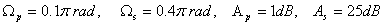程序如下：

     clear all
Wp=0.1*pi;
Ws=0.4*pi;
Ap=1;
As=25;
T=1;
Fs=1/T;
wp=Wp*Fs;
ws=Ws*Fs;
N=buttord(wp,ws,Ap,As,'s');
wc=wp/(10^(0.1*Ap)-1)^(1/2/N);
[num den]=butter(N,wc,'s');
[numd,dend]=impinvar(num,den,Fs);
w=linspace(0,pi,512);
h=freqz(numd,dend,w);
plot(w/pi,abs(h));
title('脉冲响应不变法');
%计算所设计滤波器的Ap和As
w1=[Wp,Ws];
h1=freqz(numd,dend,w1);
fprintf('Ap= %.4f\n',-20*log10(abs(h1(1))));
fprintf('As= %.4f\n',-20*log10(abs(h1(2))));


运行结果：
Ap= 0.9991
As= 30.3245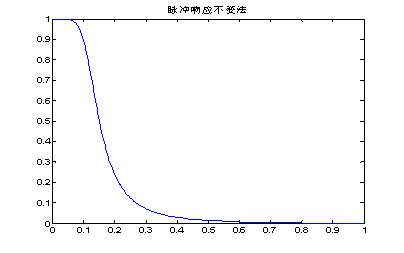图1 数字低通滤波器的幅度响应


## 2.双线性变换法

MATLAB提供bilinear(num,den,Fs)函数，可以实现利用双线性变换法将模拟滤波器转换为数字滤波器，其调用形式为：
[numd,dend]=bilinear(num,den,Fs)
式中num和den分别表示模拟滤波器系统函数H（s）的分子多项式系统和分母多项式系数，Fs=1/T。输出变量numd和dend分别表示数字滤波器系统函数H（z）的分子多项式系统和分母多项式系数。
【例6.2】利用CB1型滤波器及双线性变换法设计满足下列指标的数字高通滤波器。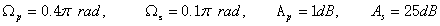程序如下：

		clear all
Wp=0.4*pi;
Ws=0.1*pi;
Ap=1;
As=25;
T=2;
Fs=1/T;
wp=(2/T)*tan(Wp/2);
ws=(2/T)*tan(Ws/2);
[N,wc]=cheb1ord(wp,ws,Ap,As,'s');
[num den]=cheby1(N,Ap,wc,'high','s');
[numd,dend]=bilinear(num,den,Fs);
w=linspace(0,pi,512);
h=freqz(numd,dend,w);
plot(w/pi,abs(h));
title('双线性变换法')
%计算所设计滤波器的Ap和As
w1=[Wp,Ws];
h1=freqz(numd,dend,w1);
fprintf('Ap= %.4f\n',-20*log10(abs(h1(1))));
fprintf('As= %.4f\n',-20*log10(abs(h1(2))));


运行结果：
Ap= 1.0000
As= 26.4153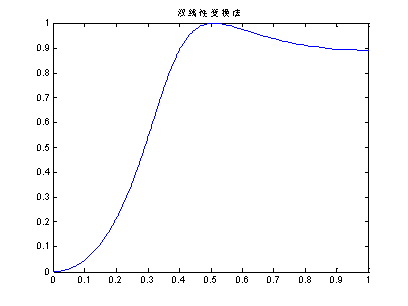图2 数字高通滤波器的幅度响应


## 3.数字滤波函数

（1）filter函数用来实现数字滤波器对数据的滤波，函数调用格式为：
y=filter(numd,dend,x)
其中，numd和dend分别为滤波器系统函数H（z）的分子和分母多项式的系数，x为滤波器的输入，y为滤波器的输出，y与x具有相同大小的向量。
（2）filtfilt函数实现零相位前后与后向结合滤波，其调用格式为：
y=filtfilt(numd,dend,x)
其中，numd和dend分别为滤波器系统函数H（z）的分子和分母多项式的系数，x为滤波器的输入，y为滤波器的输出，y与x具有相同大小的向量，这个函数实现的滤波后其输出信号与输入信号的相位一致，也就是没有改变信号波形形状。但filter函数滤波后有一些延迟，改变了信号的形状。

# 三、作业：

(1)示例代码：

clear all
Wp=0.2*pi;
Ws=0.3*pi;
Ap=1;
As=15;
T=0.01;
Fs=1/T;
wp=Wp*Fs;
ws=Ws*Fs;
N=buttord(wp,ws,Ap,As,'s');
wc=wp/(10^(0.1*Ap)-1)^(1/2/N);
[num den]=butter(N,wc,'s');
[numd,dend]=impinvar(num,den,Fs);
w=linspace(0,pi,512);
h=freqz(numd,dend,w);
plot(w/pi,abs(h));
title('脉冲响应不变法');
%计算所设计滤波器的Ap和As
w1=[Wp,Ws];
h1=freqz(numd,dend,w1);
fprintf('Ap= %.4f\n',-20*log10(abs(h1(1))));
fprintf('As= %.4f\n',-20*log10(abs(h1(2))));


（2）运行结果：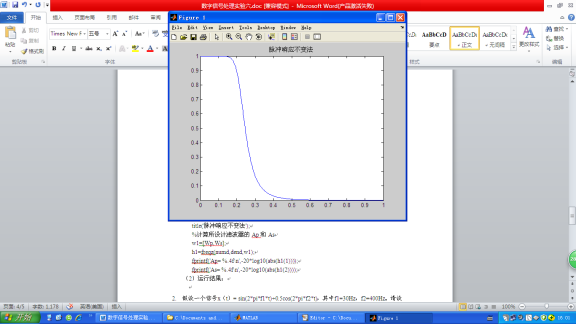2.假设一个信号x（t）= sin(2pif1t)+0.5cos(2pif2t)，其中f1=30Hz，f2=400Hz。请设计一个IIR数字滤波器，将f2滤除掉。请写出程序，并画出原信号与原信号通过滤波器的输出信号的图形。（数字低通滤波器）
（1）示例代码：

clear all
Wp=0.075*pi;
Ws=1*pi;
Ap=1;
As=25;
T=0.01;
Fs=1/T;
wp=Wp*Fs;
ws=Ws*Fs;
N=buttord(wp,ws,Ap,As,'s');
wc=wp/(10^(0.1*Ap)-1)^(1/2/N);
[num den]=butter(N,wc,'s');
[numd,dend]=impinvar(num,den,Fs);

w=linspace(0,pi,512);
h=freqz(numd,dend,w);
plot(w/pi,abs(h));
title('脉冲响应不变法');
%计算所设计滤波器的Ap和As
w1=[Wp,Ws];
h1=freqz(numd,dend,w1);
fprintf('Ap= %.4f\n',-20*log10(abs(h1(1))));
fprintf('As= %.4f\n',-20*log10(abs(h1(2))));
%信号传输
fs=800;
dt=1/fs;   %模拟信号采样间隔
f1=30;f2=400;
t=0:dt:1;
x=sin(2*pi*f1*t)+0.5*cos(2*pi*f2*t);
y=filter(numd,dend,x); % 模拟输出
L=length(x);
x1=fftshift(fft(x));
ff=(-L/2:L/2-1)*fs/L;
y1=fftshift(fft(y));
figure(2)
subplot(2,2,1)
plot(t,x);
xlabel ('(a)输入信号');
subplot(2,2,2)
plot(ff,abs(x1));
xlabel ('(b)输入信号频谱');
subplot(2,2,3)
plot(t,y);
xlabel('(c)输出信号');
subplot(2,2,4)
plot(ff,abs(y1));
xlabel ('(d)输出信号频谱'


（2）运行结果：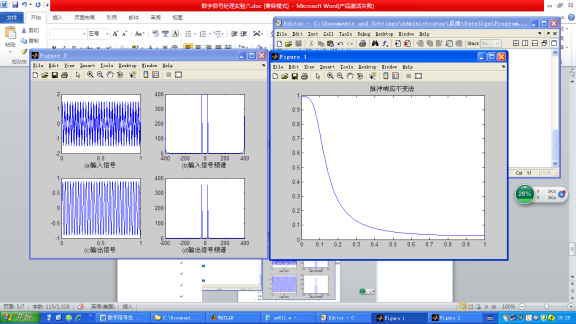3.假设一个信号x（t）= sin(2pif1t)+0.5cos(2pif2t)，其中f1=10Hz，f2=100Hz。请设计一个IIR数字滤波器能把f1滤除掉，请写出程序，并画出原信号与原信号通过滤波器的输出信号的图形。
（1）示例代码：

clear all
Wp=0.1*pi;
Ws=0.2*pi;
Ap=1;
As=25;
T=1;
Fs=1/T;
wp=(2/T)*tan(Wp/2);
ws=(2/T)*tan(Ws/2);
[N,wc]=cheb1ord(wp,ws,Ap,As,'s');
[num den]=butter(N,wc,'s');
[numd,dend]=impinvar(num,den,Fs);
w=linspace(0,pi,512);
h=freqz(numd,dend,w);
plot(w/pi,abs(h));
title('脉冲响应不变法');
%计算所设计滤波器的Ap和As
w1=[Wp,Ws];
h1=freqz(numd,dend,w1);
fprintf('Ap= %.4f\n',-20*log10(abs(h1(1))));
fprintf('As= %.4f\n',-20*log10(abs(h1(2))));
%信号传输
fs=800;
dt=1/fs;   %模拟信号采样间隔
f1=10;f2=100;
t=0:dt:1;
x=sin(2*pi*f1*t)+0.5*cos(2*pi*f2*t);
y=filter(numd,dend,x); % 模拟输出
L=length(x);
x1=fftshift(fft(x));
ff=(-L/2:L/2-1)*fs/L;
y1=fftshift(fft(y));
figure(2)
subplot(2,2,1)
plot(t,x);
xlabel ('(a)输入信号');
subplot(2,2,2)
plot(ff,abs(x1));
xlabel ('(b)输入信号频谱');
subplot(2,2,3)
plot(t,y);
xlabel('(c)输出信号');
subplot(2,2,4)
plot(ff,abs(y1));
xlabel ('(d)输出信号频谱');


（2）运行结果：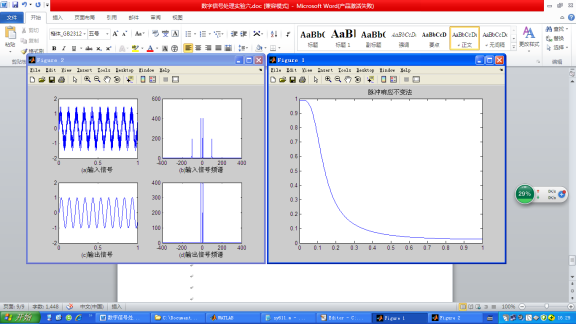4.假设一个信号x（t）=sin(2pif1t)+sin(2pif2t)+sin(2pif3*t),其中f1=200Hz，f2=1500Hz，f3=2900Hz。请设计一个IIR数字滤波器能把f2保留，请写出程序，并画出原信号与原信号通过滤波器的输出信号的图形。
（1）示例代码：

clear all
Wp=[0.2*pi 0.4*pi];
Ws=[0.1*pi 0.5*pi];
Ap=1;
As=25;
T=1;
Fs=1/T;
wp=(2/T)*tan(Wp/2);
ws=(2/T)*tan(Ws/2);
[N,wc]=cheb1ord(wp,ws,Ap,As,'s');
wc=wp/(10^(0.1*Ap)-1)^(1/2/N);
[num den]=butter(N,wc,'s');
[numd,dend]=impinvar(num,den,Fs);
w=linspace(0,pi,512);
h=freqz(numd,dend,w);
plot(w/pi,abs(h));
title('脉冲响应不变法');
%计算所设计滤波器的Ap和As
w1=[Wp,Ws];
h1=freqz(numd,dend,w1);
fprintf('Ap= %.4f\n',-20*log10(abs(h1(1))));
fprintf('As= %.4f\n',-20*log10(abs(h1(2))));
%信号传输
fs=800;
dt=1/fs;   %模拟信号采样间隔
f1=200;f2=1500;f3 = 2900;
t=0:dt:1;
x=sin(2*pi*f1*t)+sin(2*pi*f2*t)+sin(2*pi*f3*t);
y=filter(numd,dend,x); % 模拟输出
L=length(x);
x1=fftshift(fft(x));
ff=(-L/2:L/2-1)*fs/L;
y1=fftshift(fft(y));
figure(2)
subplot(2,2,1)
plot(t,x);
xlabel ('(a)输入信号');
subplot(2,2,2)
plot(ff,abs(x1));
xlabel ('(b)输入信号频谱');
subplot(2,2,3)
plot(t,y);
xlabel('(c)输出信号');
subplot(2,2,4)
plot(ff,abs(y1));
xlabel ('(d)输出信号频谱');


（2）运行结果：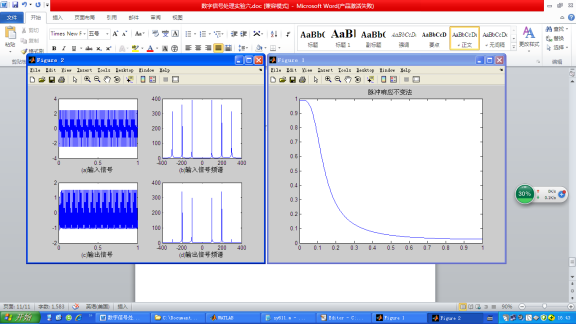# 更多相关文章点这里哦

数字信号处理----全套Matlab实验报告

通信工程(信息类，电子类，电气工程，自动化，计算机，软件工程，机电，等相关专业)全套学习指导答疑资料qq群:1007576722

展开全文算法
• 基于MATLAB GUI的IIR数字滤波器语音信号去噪处理平台的设计与实现 代码而已
• 基于MATLABIIR数字滤波器的设计基于MATLABIIR数字滤波器的设计基于MATLABIIR数字滤波器的设计
• （2）学会调用MATLAB信号处理工具箱中滤波器设计函数（或滤波器设计分析工具fdatool）设计各种IIR数字滤波器，学会根据滤波需求确定滤波器指标参数。 （3）掌握IIR数字滤波器MATLAB实现方法。 （3）通过观察滤波器...
• 2.内容：基于Matlab的fir和iir数字滤波器的设计与仿真包括matlab程序，word论文 ，PPT，程序操作视频 3.用处：用于fir和iir数字滤波器编程学习 4.指向人群：本硕博等学习教研使用,企事业简单项目方案验证参考
• 基于MATLAB GUI的IIR数字滤波器平台设计.pdf
• 它是在 GUI 的帮助下使用模数映射 (ADM) 设计 IIR（无限脉冲响应）数字滤波器的另一种方法。 图形用户界面 (GUI) 是使用图形对象（例如按钮、文本字段、滑块和菜单）构建的用户界面。 它用于设计巴特沃斯滤波器、...
• 基于matlab的IIR滤波器设计-基于matlabIIR数字滤波器设计-刘智.doc 全面的介绍IIR滤波器的设计
• 数字信号处理第四次试验：IIR数字滤波器设计及软件实现前言一、实验目的二、实验原理与方法三、实验环境四、实验内容及步骤五、实验结果截图（含分析）六、思考题 前言 为了帮助同学们完成痛苦的实验课程设计，本...

# 前言

为了帮助同学们完成痛苦的实验课程设计，本作者将其作出的实验结果及代码贴至CSDN中，供同学们学习参考。如有不足或描述不完善之处，敬请各位指出，欢迎各位的斧正！

# 一、实验目的

（1）熟悉用双线性变换法设计IIR数字滤波器的原理与方法；
（2）学会调用MATLAB信号处理工具箱中滤波器设计函数（或滤波器设计分析工具fdatool）设计各种IIR数字滤波器，学会根据滤波需求确定滤波器指标参数。
（3）掌握IIR数字滤波器的MATLAB实现方法。
（4）通过观察滤波器输入输出信号的时域波形及其频谱，建立数字滤波的概念。

# 二、实验原理与方法

设计IIR数字滤波器一般采用间接法（脉冲响应不变法和双线性变换法），应用最广泛的是双线性变换法。基本设计过程是：①先将给定的数字滤波器的指标转换成过渡模拟滤波器的指标；②设计过渡模拟滤波器；③将过渡模拟滤波器系统函数转换成数字滤波器的系统函数。MATLAB信号处理工具箱中的各种IIR数字滤波器设计函数都是采用双线性变换法。第六章介绍的滤波器设计函数butter、cheby1、cheby2和ellip可以分别被调用来直接设计巴特沃斯、切比雪夫1、切比雪夫2和椭圆模拟和数字滤波器。本实验要求读者调用如上函数直接设计IIR数字滤波器。
本实验的数字滤波器的MATLAB实现是指调用MATLAB信号处理工具箱函数filter对给定的输入信号x(n)进行滤波，得到滤波后的输出信号y(n）。

# 三、实验环境

Matlab 7.0及Matlab 2018b

# 四、实验内容及步骤

（1）调用信号产生函数mstg产生由三路抑制载波调幅信号相加构成的复合信号st，该函数还会自动绘图显示st的时域波形和幅频特性曲线，如图所示。由图可见，三路信号时域混叠无法在时域分离。但频域是分离的，所以可以通过滤波的方法在频域分离，这就是本实验的目的。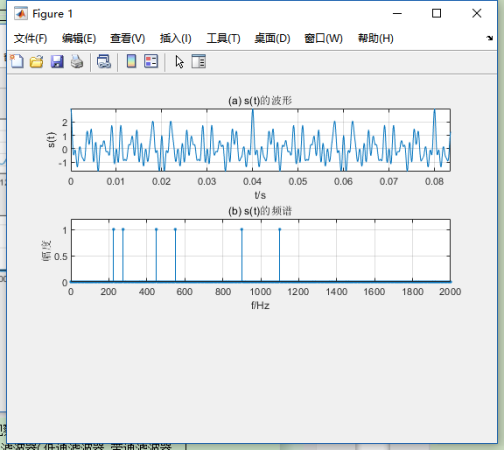（2）要求将st中三路调幅信号分离，通过观察st的幅频特性曲线，分别确定可以分离st中三路抑制载波单频调幅信号的三个滤波器（低通滤波器、带通滤波器、高通滤波器）的通带截止频率和阻带截止频率。要求滤波器的通带最大衰减为0.1dB,阻带最小衰减为60dB。
提示：抑制载波单频调幅信号的数学表示式为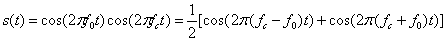其中， c o s ( 2 π f c t ) cos(2\pi f_ct) 称为载波， f c f_c 为载波频率， c o s ( 2 π f 0 t ) cos(2\pi f_0t) 称为单频调制信号， f 0 f_0 为调制正弦波信号频率，且满足 f c > f 0 f_c>f_0 。由上式可见，所谓抑制载波单频调幅信号，就是2个正弦信号相乘，它有2个频率成分：和频 f c + f 0 f_c+f_0 和差频 f c − f 0 f_c-f_0 ，这2个频率成分关于载波频率 f c f_c 对称。所以，1路抑制载波单频调幅信号的频谱图是关于载波频率 f c f_c 对称的2根谱线，其中没有载频成分，故取名为抑制载波单频调幅信号。容易看出，图中三路调幅信号的载波频率分别为250Hz、500Hz、1000Hz。如果调制信号m(t)具有带限连续频谱，无直流成分，则 s ( t ) = m ( t ) c o s ( 2 π f c t ) s(t)=m(t)cos(2\pi f_ct) 就是一般的抑制载波调幅信号。其频谱图是关于载波频率 f c f_c 对称的2个边带（上下边带），在专业课通信原理中称为双边带抑制载波(DSB-SC)调幅信号,简称双边带(DSB)信号。如果调制信号m(t)有直流成分，则 s ( t ) = m ( t ) c o s ( 2 π f c t ) s(t)=m(t)cos(2\pi f_ct) 就是一般的双边带调幅信号。其频谱图是关于载波频率 f c f_c 对称的2个边带（上下边带），并包含载频成分。
（3）编程序调用MATLAB滤波器设计函数ellipord和ellip分别设计这三个椭圆滤波器，并绘图显示其幅频响应特性曲线。
（4）调用滤波器实现函数filter，用三个滤波器分别对信号产生函数mstg产生的信号st进行滤波，分离出st中的三路不同载波频率的调幅信号 y 1 ( n ) y_1(n) y 2 ( n ) y_2(n) y 3 ( n ) y_3(n) ，并绘图显示 y 1 ( n ) y_1(n) y 2 ( n ) y_2(n) y 3 ( n ) y_3(n) 的时域波形，观察分离效果。

# 五、实验结果截图（含分析）

滤波器参数及实验程序清单
1、滤波器参数选取
观察图可知，三路调幅信号的载波频率分别为250Hz、500Hz、1000Hz。带宽（也可以由信号产生函数mstg清单看出）分别为50Hz、100Hz、200Hz。所以，分离混合信号st中三路抑制载波单频调幅信号的三个滤波器（低通滤波器、带通滤波器、高通滤波器）的指标参数选取如下：
对载波频率为250Hz的条幅信号，可以用低通滤波器分离，其指标为
带截止频率 f p f_p =280Hz，通带最大衰减 a p a_p =0.1dB；
阻带截止频率 f s f_s =450Hz，阻带最小衰减 a s a_s =60dB，
对载波频率为500Hz的条幅信号，可以用带通滤波器分离，其指标为
带截止频率 f p i f_{pi} =440Hz， f p u f_{pu} =560Hz，通带最大衰减 a p a_p =0.1dB；
阻带截止频率 f s i f_{si} =275Hz， f s u f_{su} =900Hz，阻带最小衰减 a s a_s =60dB，
对载波频率为1000Hz的条幅信号，可以用高通滤波器分离，其指标为
带截止频率 f p f_p =890Hz，通带最大衰减 a p a_p =0.1dB；
阻带截止频率 f s f_s =550Hz，阻带最小衰减 a s a_s =60dB，
说明：（1）为了使滤波器阶数尽可能低，每个滤波器的边界频率选择原则是尽量使滤波器过渡带宽尽可能宽。
（2）与信号产生函数mstg相同，采样频率Fs=10kHz。
（3）为了滤波器阶数最低，选用椭圆滤波器。
按照图4.2所示的程序框图编写的实验程序为exp4.m。

实验程序清单

%IIR数字滤波器设计及软件实现
clear;close all
Fs=10000;T=1/Fs;%采样频率
%调用信号产生函数mstg产生由三路抑制载波调幅信号相加构成的复合信号st
st=mstg;
%低通滤波器设计与实现=========================================
fp=280;fs=450;
wp=2*fp/Fs;ws=2*fs/Fs;rp=0.1;rs=60;%OF指标(低通滤波器的通、阻带边界频)
[N,wp]=ellipord(wp,ws,rp,rs);%调用ellipord计算椭圆DF阶数N和通带截止频率wp
[B,A]=ellip(N,rp,rs,wp);%调用ellip计算椭圆带通DF系统函数系数向量B和A
y1t=filter(B,A,st);%滤波器软件实现
%低通滤波器设计与实现绘图部分
figure(2);subplot(3,1,1);
myplot(B,A);%调用绘图函数myplot绘制损耗函数曲线
yt='y_1(t)';
subplot(3,1,2);tplot(y1t,T,yt);%调用绘图函数tplot绘制滤波器输出波形
%带通滤波器设计与实现=================================================
fpl=440;fpu=560;fsl=275;fsu=900;
wp=[2*fpl/Fs,2*fpu/Fs];ws=[2*fsl/Fs,2*fsu/Fs];rp=0.1;rs=60;
[N,wp]=ellipord(wp,ws,rp,rs);%调用e1lipord计算椭圆DF阶数N和通带截止频率wp
[B,A]=ellip(N,rp,rs,wp);%调用ellip计算椭圆带通DF系统函数系数向量B和A
y2t=filter(B,A,st);%滤波器软件实现
%带通滤波器设计与实现绘图部分
figure(2);subplot(3,1,1);
myplot(B,A);%调用绘图函数myplot绘制损耗函数曲线
yt='y_2(t)';
subplot(3,1,2);tplot(y2t,T,yt);axis([0,0.08,-1.2,1.2])%调用绘图函数tplot绘制滤波器输出波形
%高通滤波器设计与实现================================================
fp=890;fs=600;
wp=2*fp/Fs;ws=2*fs/Fs;rp=0.1;rs=60;%OF指标(低通滤波器的通、阻带边界频)
[N,wp]=ellipord(wp,ws,rp,rs);%调用ellipord计算椭圆DF阶数N和通带截止频率wp
[B,A]=ellip(N,rp,rs,wp,'high');%调用ellip计算椭圆带通DF系统函数系数向量B和A
y3t=filter(B,A,st);%滤波器软件实现
%高低通滤波器设计与实现绘图部分
figure(2);subplot(3,1,1);
myplot(B,A);%调用绘图函数myplot绘制损耗函数曲线
yt='y_3(t)';
subplot(3,1,2);tplot(y3t,T,yt);axis([0,0.08,-1.2,1.2])

function tplot(xn,T,yn)
n=0:length(xn)-1;
t=n*T;
plot(t,xn);
xlabel('t/s');
ylabel('yn');
axis([0,t(end),min(xn),1.2*max(xn)]);

function myplot(B,A)
[H,W]=freqz(B,A,1000);
m=abs(H);
plot(W/pi,20*log10(m/max(m)));
grid on;
xlabel('\omega/\pi');
ylabel('幅度(dB)')
axis([0,1,-80,5]);
title('损耗函数曲线');

function st=mstg
%产生信号序列向量st,并显示st的时域波形和频谱
%st=mstg返回三路调幅信号相加形成的混合信号，长度N=1600
N=1600%N为信号st的长度。
Fs=10000;T=1/Fs;Tp=N*T;%采样频率Fs=10kHz，Tp为采样时间
t=0:T:(N-1)*T;k=0:N-1;f=k/Tp;
fc1=Fs/10;%第1路调幅信号的载波频率fc1=1000Hz,
fm1=fc1/10;%第1路调幅信号的调制信号频率fm1=100Hz
fc2=Fs/20;%第2路调幅信号的载波频率fc2=500Hz
fm2=fc2/10;%第2路调幅信号的调制信号频率fm2=50Hz
fc3=Fs/40;%第3路调幅信号的载波频率fc3=250Hz,
fm3=fc3/10;%第3路调幅信号的调制信号频率fm3=25Hz
xt1=cos(2*pi*fm1*t).*cos(2*pi*fc1*t);%产生第1路调幅信号
xt2=cos(2*pi*fm2*t).*cos(2*pi*fc2*t);%产生第2路调幅信号
xt3=cos(2*pi*fm3*t).*cos(2*pi*fc3*t);%产生第3路调幅信号
st=xt1+xt2+xt3;%三路调幅信号相加
fxt=fft(st,N);%计算信号st的频谱
subplot(3,1,1)
plot(t,st);grid;xlabel('t/s');ylabel('s(t)');
axis([0,Tp/8,min(st),max(st)]);title('(a)s(t)的波形')
subplot(3,1,2)
stem(f,abs(fxt)/max(abs(fxt)),'.');grid;title('(b)s(t)的频谱')
axis([0,Fs/5,0,1.2]);
xlabel('f/Hz');ylabel('幅度')


实验程序运行结果及分析讨论
实验4程序运行结果如图所示。由图可见，三个分离滤波器指标参数选取正确，算耗函数曲线达到所给指标。分离出的三路信号y1(n)，y2(n)和y3(n)的波形是抑制载波的单频调幅波。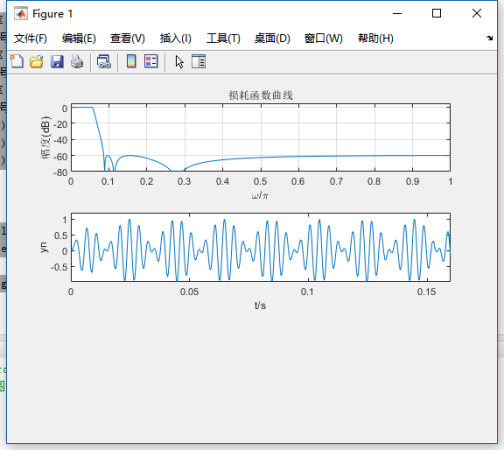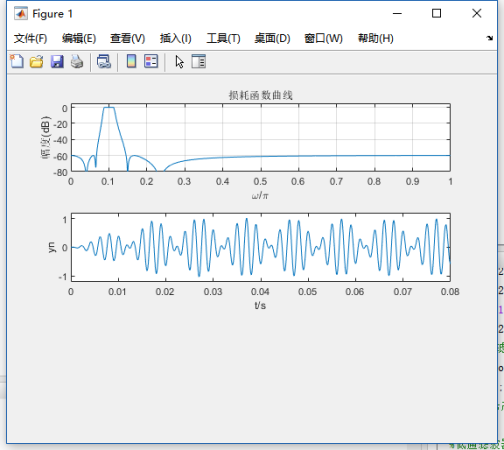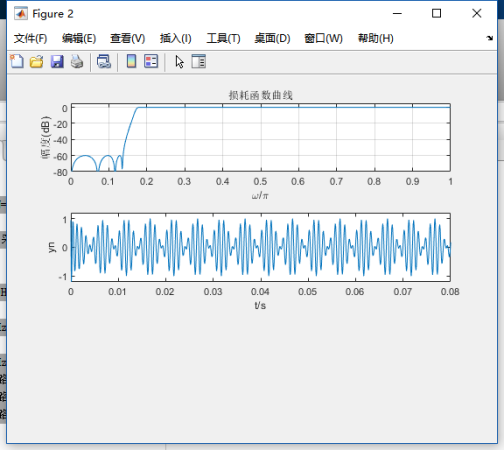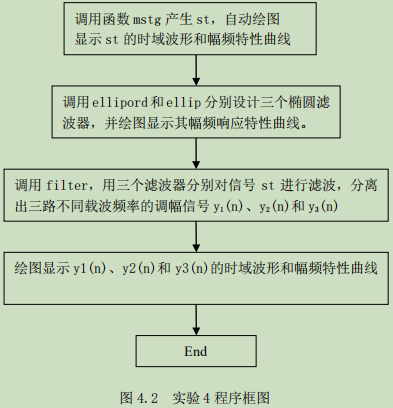# 六、思考题

（1）请阅读信号产生函数mstg，确定三路调幅信号的载波频率和调制信号频率。
（2）信号产生函数mstg中采样点数N=800，对st进行N点FFT可以得到6根理想谱线。如果取N=1000，可否得到6根理想谱线？为什么？N=2000呢？请改变函数mstg中采样点数N的值，观察频谱图验证您的判断是否正确。
（3）修改信号产生函数mstg，给每路调幅信号加入载波成分，产生调幅（AM）信号，重复本实验，观察AM信号与抑制载波调幅信号的时域波形及其频谱的差别。
提示：AM信号表示式： s ( t ) = [ 1 + c o s ( 2 π f 0 t ) ] c o s ( 2 π f c t ) s(t)=[1+cos(2\pi f_0t)]cos(2\pi f_ct)
答：（1）观察图可知，三路调幅信号的载波频率分别为250Hz、500Hz、1000Hz。带宽（也可以由信号产生函数mstg清单看出）分别为50Hz、100Hz、200Hz。所以，分离混合信号st中三路抑制载波单频调幅信号的三个滤波器（低通滤波器、带通滤波器、高通滤波器）的指标参数选取如下：
对载波频率为250Hz的条幅信号，可以用低通滤波器分离，其指标为
带截止频率 f p f_p =280Hz，通带最大衰减 a p a_p =0.1dB；
阻带截止频率 f s f_s =450Hz，阻带最小衰减 a s a_s =60dB，
对载波频率为500Hz的条幅信号，可以用带通滤波器分离，其指标为
带截止频率 f p i f_{pi} =440Hz， f p u f_{pu} =560Hz，通带最大衰减 a p a_p =0.1dB；
阻带截止频率 f s i f_{si} =275Hz， f s u f_{su} =900Hz，阻带最小衰减 a s a_s =60dB，
对载波频率为1000Hz的条幅信号，可以用高通滤波器分离，其指标为
带截止频率 f p f_p =890Hz，通带最大衰减 a p a_p =0.1dB；
阻带截止频率 f s f_s =550Hz，阻带最小衰减 a s a_s =60dB，
（3）因为信号st是周期序列，谱分析时要求观察时间为整数倍周期。所以，
本题的一般解答方法是，先确定信号st的周期，在判断所给采样点数N对应的观察时间Tp=NT是否为st的整数个周期。但信号产生函数mstg产生的信号st共有6个频率成分，求其周期比较麻烦，故采用下面的方法解答。
分析发现，st的每个频率成分都是25Hz的整数倍。采样频率Fs=10kHz=25×400Hz，即在25Hz的正弦波的1个周期中采样400点。所以，当N为400的整数倍时一定为st的整数个周期。因此，采样点数N=800和N=2000时，对st进行N点FFT可以得到6根理想谱线。如果取N=1000，不是400的整数倍，不能得到6根理想谱线。

展开全文• 　学会调用MATLAB信号处理工具箱中滤波器设计函数设计各种IIR数字滤波器，学会根据滤波需求确定滤波器指标参数。　掌握IIR数字滤波器MATLAB实现方法。　通过观察滤波器输入输出信号的时域波形及其频谱，建立数字...
• 长冲激响应(IIR)数字滤波器和有限长冲激响应(FIR)数字滤波器。实现IIR滤波 器的阶次较低，所用的存储单元较少，效率高，精度高，而且能够保留一些模拟 滤波器的优良特性，因此应用很广。Matlab软件以矩阵运算为...
• （1） 1设计FIR滤波器：先把期望数字滤波器的指标通过预畸变转化成模拟滤波器的指标，再用MATLAB文件得到模拟滤波器的传输函数，再用双线性变换法得到数字滤波器的传输函数。 （2） 2设计IIR滤波器：先通过数字...
• （2）学会调用MATLAB信号处理工具箱中滤波器设计函数（或滤波器设计分析工具fdatool）设计各种IIR数字滤波器，学会根据滤波需求确定滤波器指标参数。 （3）掌握IIR数字滤波器MATLAB实现方法。 （3）通过观察...
• 《毕业论文：IIR数字滤波器设计及其MATLAB实现.doc》由会员分享，可免费在线阅读全文，更多与《(终稿)毕业论文：IIR数字滤波器设计及其MATLAB实现.doc(OK版)》相关文档资源请在帮帮文库(www.woc88.com)数亿文档库存...
• 本课题研究的是在MATLAB软件下IIR数字滤波器的设计，以及在语音信号处理方面的应用。先简单描述了MATLAB软件的特点，介绍了巴特沃斯低通滤波器的设计步骤。然后叙述了IIR数字滤波器对语音信号处理的应用，包括语音...
• 这是一个用于设计 iir 滤波器matlab 程序，可以修改该程序以获得其他类型的滤波器
• 数字信号处理实验十五 IIR 数字滤波器三种结构形式的软件实现 一．实验目的 1. 掌握IIR 数字滤波器设计的方法； 2. 掌握IIR 数字滤波器直接型、级联型和并联型的基本特点并根据给定的传递函数形式正 确选择是否采用...数字信号处理
• IIR数字滤波器MATLAB中的设计与实现.pdf
• 2.学会调用MATLAB信号处理工具箱中滤波器设计函数（或滤波器设计分析工具FDATool）设计各种IIR数字滤波器，学会根据滤波需求确定滤波器指标参数。 3.掌握IIR数字滤波器MATLAB实现方法。 4.通过观察滤波器输入、...
• 本次数字滤波器设计方法是基于MATLAB的数字滤波器的设计，是用学过的数字信号理论为依据，用MATLAB代码来实现的。通过IIR数字滤波器的设计过程，说明如何利用MATLAB来完成数字滤波器的设计。...

# iir数字滤波器matlab程序matlab 订阅